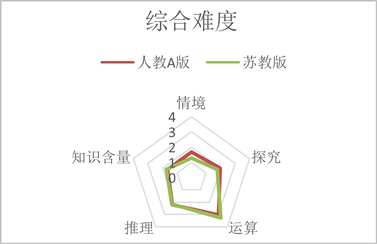#### 期刊菜单

A Comparative Study of the Content of the Number Series of High School Mathematics Textbooks of the PEP A Edition and the Jiangsu Education Edition
DOI: 10.12677/CES.2023.118327, PDF , HTML, XML, 下载: 113  浏览: 213

Abstract: According to the new curriculum standards, different versions of high school mathematics text-books have been compiled in various places, and different versions of textbooks have different characteristics, and the number series is an important part of the high school mathematics teaching content, so it is necessary to compare and study the number series chapters of different versions of the textbook. This paper takes the number series chapters of the 2019 PEP A and Jiangsu Education Edition high school mathematics textbooks as the research object, and compares and analyzes the two versions of the textbooks from the three parts of column setting, knowledge point selection, example problems and exercise difficulty to obtain the writing characteristics of the two versions of the textbooks and suggestions for the flexible use of the two versions of the textbooks.

1. 问题提出

2. 研究设计

2.1. 研究对象

2.2. 研究模型Table 1. Difficulty factor level division table

3. 两种教材数列部分的比较

3.1. 栏目设置的比较Table 2. Two versions of the textbook series chapter column setting

3.2. 知识点选取的比较Table 3. The two versions of the textbook list of knowledge points and their distribution table

3.3. 例题、习题难度的比较Table 4. Statistical table of difficulty factors of example and exercise in the two versions of textbooksTable 5. Statistical table of weighted average comprehensive difficulty of example and exercise in the two versions of textbooksFigure 1. Comprehensive difficulty radar chart of series examples and exercises in the two textbooks

4. 建议与启示

4.1. 强调数列与函数的联系，渗透数学思想方法

4.2. 注重数学史的渗透，关注数学知识的形成过程

4.3. 加强信息技术在数列中的应用，增强学生信息素养

4.4. 合理使用例题、习题

  王子扬. 中英高中数学教材比较研究[D]: [硕士学位论文]. 上海: 华东师范大学, 2021.  张婧婧. 人教A版与苏教版高中数学教材数列部分的比较研究[J]. 数学教学研究, 2014, 33(10): 24-27.  中华人民共和国教育部. 普通高中数学课程标准(2017年版2020年修订) [M]. 北京: 人民教育出版社, 2020.  鲍建生. 中英两国初中数学期望课程综合难度的比较[J]. 全球教育展望, 2002, 31(9): 48-52.  朱琛, 唐恒钧, 徐元根. 中澳初中数学教科书习题情境的比较分析——以“统计与概率”领域为例[J]. 现代中小学教育, 2018, 34(1): 91-95.  马超. 高中数学教材数列内容的比较研究[D]: [硕士学位论文]. 苏州: 苏州科技大学, 2021.  曾小毛. 2019人教A版、北师大版和湘教版高中数学教材数列内容的比较研究[D]: [硕士学位论文]. 重庆: 西南大学, 2021.  张日新. 高中数学新旧教材数列内容的比较研究[D]: [硕士学位论文]. 石家庄: 河北师范大学, 2022.